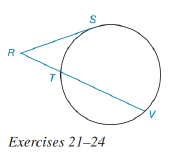Chapter 6.3, Problem 24EElementary Geometry For College St...

7th Edition
Alexander + 2 others
ISBN: 9781337614085

Solutions

Chapter
SectionElementary Geometry For College St...

7th Edition
Alexander + 2 others
ISBN: 9781337614085
Textbook Problem

In the figure for Exercises 21 to 24, R S ¯ is tangent to the circle at S. See Theorem 6.3.7.Given: R T = 1 2 ⋅ R S     a n d     T V = 9 Find: RT.To determine

To find:

The value of RT

Explanation

Given that, RT=12RSandTV=9

The diagrammatic representation is given below,

Theorem:

If a tangent segment and a secant segment are drawn to a circle from an external point, then the square of the length of the tangent equals the product of the length of the secant with the length of its external segment.

By using the theorem to get the following,

RS2=RVRT

We know that

RV=RT+TVRV=12RS+9

Substitute the values RT=12RSandRV=12RS+9 in the above equation to get the following,

Still sussing out bartleby?

Check out a sample textbook solution.

See a sample solution

The Solution to Your Study Problems

Bartleby provides explanations to thousands of textbook problems written by our experts, many with advanced degrees!

Get Started

Find more solutions based on key concepts